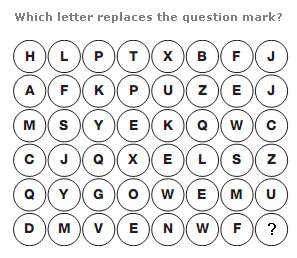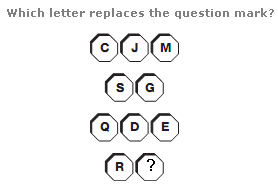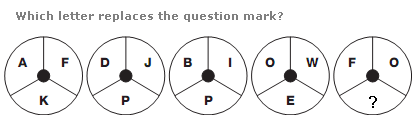# Puzzles - Missing letters puzzles

### Exercise :: Missing letters puzzlesAnswer : O Explanation : In each row, letters follow the alphabetic sequence, in steps of 4 letters each time for the first row, 5 letters for the second, then 6, 7 etc.Answer : H Explanation : In each row of the diagram, the numerical values of the letters add up to 26.Answer : X Explanation : In each circle, start with the top left segment, and move clockwise around the other segments. The value of each letter increases by 5 for the first circle, 6 for the next, then 7, 8 and 9.